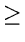Next:2.7 Programming AssignmentsUp:2. ListsPrevious:2.5 To Probe Further

# 2.6 Problems

1.
A linked list has exactly n nodes. The elements in these nodes are selected from the set {0, 1,..., n}. There are no duplicates in the list. Design an O(n) worst case time algorithm to find which one of the elements from the above set is missing in the given linked list.
2.
Write a procedure that will reverse a linked list while traversing it only once. At the conclusion, each node should point to the node that was previously its predecessor: the head should point to the node that was formerly at the end, and the node that was formerly first should have a null link.
3.
How would one implement a queue if the elements that are to be placed on the queue are arbitrary length strings? How long does it take to enqueue a string?
4.
Let A be an array of size n, containing positive or negative integers, with A < A <...< A[n]. Design an efficient algorithm (should be more efficient than O(n)) to find an isuch that A[i] = i provided such an i exists. What is the worst case computational complexity of your algorithm ?
5.
Consider an array of size n. Sketch an O(n) algorithm to shift all items in the array k places cyclically counterclockwise. You are allowed to use only one extra location to implement swapping of items.
6.
In some operating systems, the least recently used (LRU) algorithm is used for page replacement. The implementation of such an algorithm will involve the following operations on a collection of nodes.
• use a node.
• replace the LRU node by a new node.
Suggest a good data structure for implementing such a collection of nodes.
7.
A queue Q contains the items a1, a2,..., an, in that order with a1 at the front and an at the back. It is required to transfer these items on to a stack S (initially empty) so that a1 is at the top of the stack and the order of all other items is preserved. Using enqueue and dequeue operations for the queue and push and pop operations for the stack, outline an efficient O(n) algorithm to accomplish the above task, using only a constant amount of additional storage.
8.
A queue is set up in a circular array C[0..n - 1] with front and rear defined as usual. Assume that n - 1 locations in the array are available for storing the elements (with the other element being used to detect full/empty condition). Derive a formula for the number of elements in the queue in terms of rear, front, and n.
9.
Let p1p2...pn be a stack-realizable permutation of 12...n. Show that there do not exist indices i < j < k such that pj < pk < pi.
10.
Write recursive algorithms for the following problems:
(a)
Compute the number of combinations of n objects taken m at a time.
(b)
(c)
Reverse an array.
(d)
Binary search on an array of size n.
(e)
Compute gcd of two numbers n and m.
11.
Consider the following recursive definition:
 g(i, j) = i (j = 1) (2.1) g(i, j) = j (i = 1) (2.2) g(i, j) = g(i - 1, j) + g(i, j - 1) else (2.3)

Design an O(mn) iterative algorithm to compute g(m, n) where m and n are positive integers.

12.
Consider polynomials of the form:
p(x) = c1xe1 + c2xe2...c1xen;

where e1 > e2...> en0. Such polynomials can be represented by a linked lists in which each cell has 3 fields: one for the coefficient, one for the exponent, and one pointing to the next cell. Write procedures for
(a)
differentiating,
(b)
integrating,
(c)
(d)
multiplying
such polynomials. What is the running time of these procedures as a function of the number of terms in the polynomials?
13.
Suppose the numbers 1, 2, 3, 4, 5 and 6 arrive in an input stream in that order. Which of the following sequences can be realized as the output of (1) stack, and (2) double ended queue?
a)
1 2 3 4 5 6
b)
6 5 4 3 2 1
c)
2 4 3 6 5 1
d)
1 5 2 4 3 6
e)
1 3 5 2 4 6Next:2.7 Programming AssignmentsUp:2. ListsPrevious:2.5 To Probe Further
eEL,CSA_Dept,IISc,Bangalore